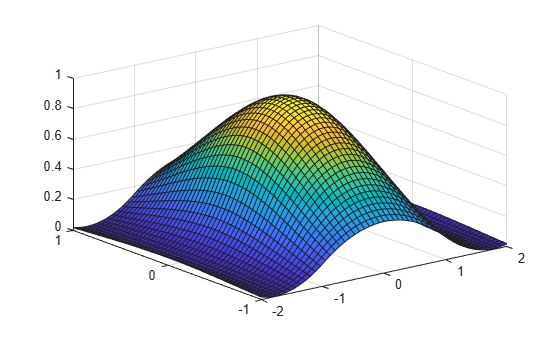# spap2

Least-squares spline approximation

## Syntax

``spline = spap2(knots,k,x,y) ``
``spap2(l,k,x,y) ``
``spline = spap2(...,x,y,w) ``
``spap2({knorl1,...,knorlm},k,{x1,...,xm},y) ``
``spap2({knorl1,...,knorlm},k,{x1,...,xm},y,w) ``

## Description

example

````spline = spap2(knots,k,x,y) ` returns the B-form of the spline f of order `k` with the given knot sequence `knots` for which(*) y(:,j) = f(x(j)), all j in the weighted mean-square sense, meaning that the sum $\sum _{j}w\left(j\right)|y\left(:,j\right)-f\left(x\left(j\right)\right){|}^{2}$is minimized, with default weights equal to 1. The data values `y(:,j)` can be scalars, vectors, matrices, or ND-arrays, and |z|2 is the sum of the squares of all the entries of z. Data points with the same site are replaced by their average.If the sites `x` satisfy the Schoenberg-Whitney conditionsthen there is a unique spline of the given order and knot sequence satisfying (*) exactly. No spline is returned unless (**) is satisfied for some subsequence of `x`.```
````spap2(l,k,x,y) `, with `l` a positive integer, returns the B-form of a least-squares spline approximant, but with the knot sequence chosen for you. The knot sequence is obtained by applying `aptknt` to an appropriate subsequence of `x`. The resulting piecewise-polynomial consists of `l` polynomial pieces and has `k-2` continuous derivatives. If you feel that a different distribution of the interior knots might do a better job, follow this up withsp1 = spap2(newknt(spline),k,x,y)); ```
````spline = spap2(...,x,y,w) ` lets you specify the weights `w` in the error measure (given above). `w` must be a vector of the same size as `x`, with nonnegative entries. All the weights corresponding to data points with the same site are summed when those data points are replaced by their average.```
````spap2({knorl1,...,knorlm},k,{x1,...,xm},y) ` provides a least-squares spline approximation to gridded data. Here, each `knorli` is either a knot sequence or a positive integer. Further, `k` must be an `m`-vector, and `y` must be an (`r+m`)-dimensional array, with `y(:,i1,...,im)` the datum to be fitted at the `site` `[x{1}(i1),...,x{m}(im)]`, all `i1`, ..., `im`. However, if the spline is to be scalar-valued, then, in contrast to the univariate case, `y` is permitted to be an `m`-dimensional array, in which case `y(i1,...,im)` is the datum to be fitted at the `site` `[x{1}(i1),...,x{m}(im)]`, all `i1`, ..., `im`. ```
````spap2({knorl1,...,knorlm},k,{x1,...,xm},y,w) ` also lets you specify the weights. In this `m`-variate case, `w` must be a cell array with `m` entries, with `w{i}` a nonnegative vector of the same size as `xi`, or else `w{i}` must be empty, in which case the default weights are used in the `i`th variable.```

## Examples

collapse all

This example shows how to compute the least-squares approximation to the data `x`, `y`, by cubic splines with two continuous derivatives, basic interval [`a`..`b`], and interior breaks `xi`, provided `xi` has all its entries in `(a..b)` and the conditions (**) are satisfied.

```sp = spap2(augknt([a,xi,b],4),4,x,y) ```

In that case, the approximation consists of `length(xi)+1` polynomial pieces. If you only want to get a cubic spline approximation consisting of `l` polynomial pieces, use instead

```sp = spap2(l,4,x,y); ```

If the resulting approximation is not satisfactory, try using a larger `l`. Else use

```sp = spap2(newknt(sp),4,x,y); ```

for a better distribution of the knot sequence. Repeat this process multiple times to increase the fidelity of the distribution.

As another example, `spap2(1,2,x,y);` provides the least-squares straight-line fit to data `x`,`y`, while

```w = ones(size(x)); w([1 end]) = 100; spap2(1,2,x,y,w); ```

forces that fit to come very close to the first data point and to the last.

This example shows how to create a bivariate function, and compute and plot its least-square approximation.

Generate the data for the approximation and the bivariate function.

```x = -2:.2:2; y=-1:.25:1; [xx, yy] = ndgrid(x,y); z = exp(-(xx.^2+yy.^2)); ```

Compute the least-square approximation and plot it.

```sp = spap2({augknt([-2:2],3),2},[3 4],{x,y},z); fnplt(sp)```## Input Arguments

collapse all

Knot sequence of the spline, specified as a nondecreasing vector.

Order of the spline f, specified as a scalar.

Number of polynomial pieces of the resulting piecewise-polynomial, specified as a positive integer.

Weights in the error measure, specified as a nonnegative vector of the same size as `x`.

Data sites of data values `y` to be fit, specified as a vector or as a cell array for multivariate data. Spline f is created with knots at each data site `x` such that f(`x(j)`) = `y(:,j)` for all values of `j`.

For multivariate, gridded data, you can specify `x` as a cell array that specifies the data site in each variable dimension: f(`x1(i),x2(j),...xn(k)`) = `y(:,i,j,...,k)`.

Data values to fit during creation of the spline, specified as a vector, matrix, or array. Data values `y(:,j)` can be scalars, matrices, or n-dimensional arrays. Data values given at the same data site `x` are averaged.

Data Types: `single` | `double`

## Output Arguments

collapse all

Spline, returned as a structure with these fields.

Form of the spline, returned as `B-`. `B-` indicates it is given in B-form.

Knot positions of the spline, returned as a vector or as a cell array of vectors for multivariate data. Vectors contain strictly increasing elements that represent the start and end of each of the intervals over which the polynomial pieces are defined.

Coefficients of polynomials for each piece, returned as a matrix or as an array for multivariate data.

Number of polynomial pieces describing the spline, returned as a scalar or as a vector of numbers of pieces in each variable for multivariate data.

Order of the polynomial function describing each polynomial piece of the spline, returned as a scalar or as a vector containing the order in each variable for multivariate data.

Dimensionality of the target function, returned as a scalar.

## Algorithms

`spcol` is called on to provide the almost block-diagonal collocation matrix (Bj,k(xi)), and `slvblk` solves the linear system (*) in the (weighted) least-squares sense, using a block QR factorization.

Gridded data are fitted, in tensor-product fashion, one variable at a time, taking advantage of the fact that a univariate weighted least-squares fit depends linearly on the values being fitted.

## Version History

Introduced before R2006a